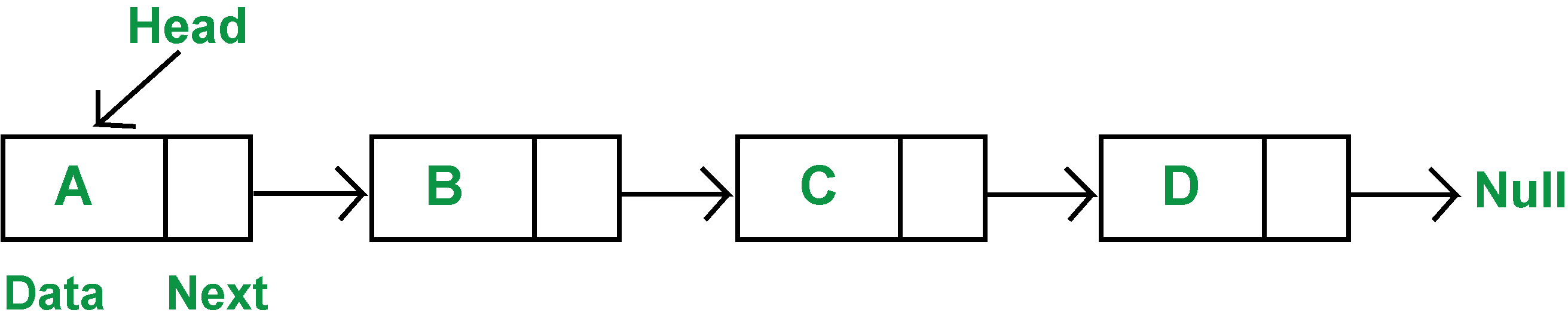# Implement Linked List in Java using Node class

How to implement a linked list in java using node class

Hey Folks,
I am back another tutorial of data structure. In this tutorial, we will learn how to implement a linked list in java using node class. It can also be done by importing class linked list from the library. But in this tutorial, we will learn to hard code the program.

## Implementing Linked List in Java using Node Class

Firstly we create a class named Node. Every node consists of an address of the next element and its value. But the last node has null stored at its address as it is the last element.

This is how one node is connected to the other node. So we create a node class and it consists of two declarations. one is integer type data and the other is Node type next variable.Creating Node.java file

```public class Node
{
int data;
Node next;
}
```

The above code creates a block of memory. Where a part of it stores the address of next data and other part stores data.

```public class Linkedlist
{

public void insert(int data)
{
Node node=new Node();
node.data=data;
node.next=null;

{
}
else
{
while(n.next!=null)
{
n=n.next;
}
n.next=node;
}
}

public void delete(int index)
{
if(index==0)
{
}
else
{
Node n1=null;
for(int i=0;i<index-1;i++)
{
n=n.next;
}
n1=n.next;
n.next=n1.next;
System.out.println("n1" + n1.data);
}
}
public void show()
{

while(node.next!=null)
{
System.out.println(node.data);
node=node.next;
}
System.out.println(node.data);
}
public void insertAtStart(int data)
{
Node node=new Node();
node.data=data;
}
public void insertAt(int index,int data)
{
Node node=new Node();
node.data=data;

for(int i=0;i<index-1;i++)
{
n=n.next;
}
node.next=n.next;
n.next=node;
}
}

```

For inserting the node at the end we simply copy the address of the node to be added to the last node. And we make the newly added node as null. We can also add an element at the start. We replace the head node with a new node. And we put the address of the next node in our head node.

We can also add an element at the index of your choice with insertAt function. For deleting a node we can also write n.next=n.next.next. It automatically deletes an adjacent node. n.next represent the address of node and n.data gives us data stored in it.

Show function print data of the node until we encounter null value. Insert functions work is to add a node. It reaches to the position where we want to add the node by .next and adds.

Creating Runner.java file

```//import java.util.LinkedList;

public class Runner {

public static void main(String[] args)
{
list.insert(18);
list.insert(7);
list.insert(15);
list.insertAtStart(25);
list.insertAt(2,55);
list.delete(2);

list.show();
}

}
```
```Output:
18
7
55
15

```

Runner class is basically our driver class which connects all our files. It calls all the functions and resulted is printed here.

We make an object of LinkedList type named list. Then we call function one by one like object.functionName(value).
1.Priyanshu says:
2.Utkarsh Tiwari says: